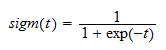StatLect

Conditional models

This lecture introduces conditional probability models, a class of statistical models in which sample data are divided into input and output data and the relation between the two kind of data is studied by modelling the conditional probability distribution of the outputs given the inputs. This is in contrast to unconditional models (sometimes also called generative models) where the data is studied by modelling the joint distribution of inputs and outputs.Introduction

Before introducing conditional models, let us review the main elements of a statistical model (see the lecture entitled Statistical inference):

1. there is a sample, which can be regarded as a realization of a random vector(for example,could be a vector collecting the realizations of some independent random variables);

2. the joint distribution function of the sample, denoted by, is not known exactly;

3. the sampleis used to infer some characteristics of;

4. a model foris used to make inferences, where a model is simply a set of joint distribution functions to whichis assumed to belong.

In a conditional model, the sampleis partitioned into inputs and outputs: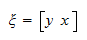wheredenotes the vector of outputs andthe vector of inputs. The object of interest is the conditional distribution function of the outputs given the inputs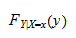and specifying a conditional model means specifying a set of conditional distribution functions to whichis assumed to belong.

In other words, in a conditional model, the problem of model specification is simplified by narrowing the focus of the statistician's attention on the conditional distribution of the outputs and by ignoring the distribution of the inputs. This can be seen, for example, in the case in which both inputs and outputs are continuous random variables. In such a case, specifying an unconditional model is equivalent to specifying a joint probability density function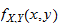for the inputs and the outputs. But a joint density can be seen as the product of a marginal and a conditional density:So, in an unconditional model we explicitly or implicitly specify both the marginal probability density functionand the conditional probability density function. On the other hand, in a conditional model, we specify only the conditionaland we leave the marginalunspecified.

Terminology

This section presents some of the terminology that is often used when dealing with conditional models.

Regression and classification

The following distinction is often made, especially in the field of machine learning:

1. if the output is a continuous random variable, then a conditional model is called a regression model;

2. if the output is a discrete random variable, taking finitely many values (typically few), then a conditional model is called a classification model.

Inputs

The input variables are often called:

• predictors

• independent variables

• features

• explanatory variables

• regressors (in the context of regression models)

Outputs

The output variables are often called:

• predictands

• dependent variables

• target variables

• response variables

• regressands (in the context of regression models)

Examples

The following subsections introduce some examples of conditional models.

Linear regression model

The linear regression model is probably the oldest, best understood and most widely used conditional model. In the linear regression model, the response variablesare assumed to be a linear function of the inputs: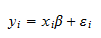whereis any observation from the sample,is a scalar output,is avector of inputs,is avector of constants (called regression coefficients) andis an unobservable random variable that adds noise to the linear relationship between inputs and outputs.

A linear regression model is speficied by making assumptions about the error term. For example,is often assumed to have a normal distribution with zero mean and to be independent of. In such a case, we have that, conditional on the inputs, the outputhas a normal distribution with mean. As a consequence, the conditional density ofiswhereis the variance of.

The parametersandare usually unknown and need to be estimated. So, we have a different conditional distribution for each of the values ofandthat are deemed plausible by the statistician before observing the sample. The set of all these conditional distributions (associated to the different parameters) constitutes the conditional model for.

In the logistic classification model, the response variableis a Bernoulli random variable: it can take only two values, eitheror. It is assumed that the conditional probability mass function ofis a non-linear function of the inputs: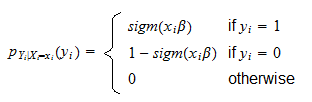whereis avector of inputs,is avector of constants andis the logistic function defined by